Courses

# Test: Solubility Product

## 15 Questions MCQ Test Chemistry for JEE | Test: Solubility Product

Description
This mock test of Test: Solubility Product for JEE helps you for every JEE entrance exam. This contains 15 Multiple Choice Questions for JEE Test: Solubility Product (mcq) to study with solutions a complete question bank. The solved questions answers in this Test: Solubility Product quiz give you a good mix of easy questions and tough questions. JEE students definitely take this Test: Solubility Product exercise for a better result in the exam. You can find other Test: Solubility Product extra questions, long questions & short questions for JEE on EduRev as well by searching above.
QUESTION: 1

### . What is the molar solubility (s) of Ba3(PO4)2 in terms of Ksp?

Solution:

Ba3(PO4)2 ⇌ 3Ba+2  (2PO4)-3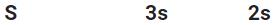Ksp =  [Ba+2>]3 [(PO4)-3]2
[3s]3[2s]2
Ksp = 108s5
Or s = (Ksp/108)

QUESTION: 2

### What is the Ksp expression for the salt PbI2?

Solution:

The correct answer is Option D.
PbI2 ‡Œ Pb2+ + 2I
or,
Ksp = [Pb2+][2I]2

QUESTION: 3

### The solubility product expression for tin(II) hydroxide, Sn(OH)2, is

Solution: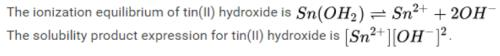QUESTION: 4

The [Ag+(aq)] = 10-5 in a solution .The [Cl(aq)] to precipitate AgCl having Ksp of 1.8×1010 M2 is — M

Solution:

For precipitation, Qrkn > Ksp, then tto establish equilibrium, ions will combine to give molecule as ppt.
So applying the above concept,
1.8×10-10 < [Ag+][Cl-]
1.8×10-10 < 10-5 × [Cl-]
Or [Cl-] > 1.8×10-5
By seeing the option, only option d satisfies this condtion.

QUESTION: 5

The molar solubility of PbBr2 is 2.17 x 10-3 M at a certain temperature. Calculate Kspfor PbBr2

Solution:

Molar solubility(s) for PbBr2 =  2.17×10-3 M
PbBr2   ⇌   Pb+2 + 2Br-
s
s          2s
Ksp = [Pb+2][Br-]2
= [s][s]2
= 4s3  =  4(2.17×10-3)3
= 4.1×10-8

QUESTION: 6

What is the correct equilibrium expression (Ksp) for the reaction below:
Ca3(PO4)2(s)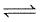3Ca2+(aq) + 2PO42-(aq)

Solution:
QUESTION: 7

What is the Ksp expression for lead (II) chloride?

Solution:

PbCl⇌ Pb2+ + 2Cl– or, Ksp = [Pb2+][Cl]2

QUESTION: 8

Solubility of BaCl2 if Ksp is 10-6 at 25°C is

Solution:

The correct answer is Option B.
BaCl₂ ⇔ Ba²⁺ + 2Cl⁻
Ksp = (s)(2s)² = 4s³
Given, 4s³ = 10⁻⁶
⇒s³ = 0.25 ⨯ 10⁻⁶
⇒s = (0.25)³ ⨯ 10⁻²
⇒s = 0.629 ⨯ 10⁻²
⇒s = 6.3 ⨯ 10⁻³

QUESTION: 9

When in a saturated solution of NaCl, HCl is passed, pure precipitate of NaCl is formed. This is due to the fact:

Solution:

In saturated solution of NaCl,equillibrium is established between ions and unionised NaCl. When HCl gas is passed through saturated solution of NaCl,it is completely dissociated to form H+ and Cl- ions. Cl- ion is common to both electrolyte ,hence Cl- ion concentration in solution increases.

QUESTION: 10

Solubility product is defined as

Solution:

Definition of Solubility Product, K.A substance's solubility product, Ksp, is the mathematical product of its dissolved ion concentrations raised to the power of their stoichiometric coefficients. Solubility products are relevant when a sparingly soluble ionic compound releases ions into solution.

QUESTION: 11

The solubility product expression for silver(I) sulphide, using x to represent the molar concentration of silver(I) and y to represent the molar concentration of sulphide, is formulated as:

Solution:

The correct answer is Option C.
The ionization equilibrium of silver (I) sulfide is
Ag2S⇌2Ag+ +S2
The solubility product expression for silver (I) sulfide is KSP[Ag+]2 [S2].
But [Ag+]2 = x and [S2−] = y.
Hence the expression for the solubility product becomes KSP[Ag+]2 [S2−] = x2y.

QUESTION: 12

Consider the following solubility data for various chromates at 25°C.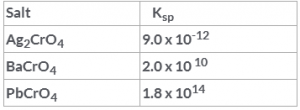Solution:

The salt with more Ksp is more soluble in water. Hence BaCrO4 is more soluble.

QUESTION: 13

A solution is 0.0010 M in both Ag+ and Au+. Some solid NaCl is added slowly until the solid AgCl just begins to precipitate. What is the concentration of Au+ ions at this point? Ksp for AgCl = 1.8 x 10-10 and for AuCl = 2.0 x 10-13.

Solution: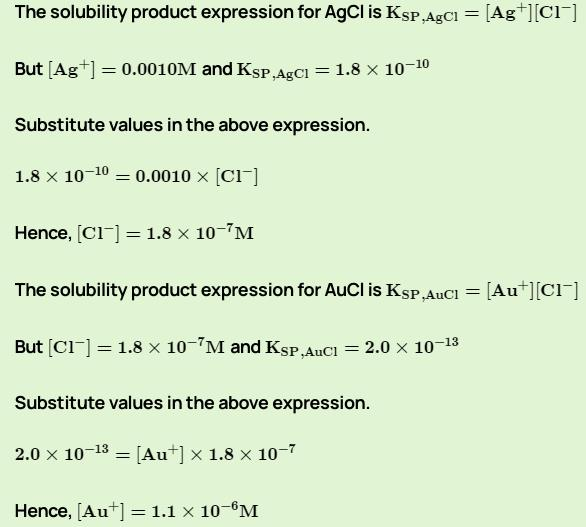QUESTION: 14

Precipitation requires

Solution:

PRECIPITATION: Precipitation occurs when tiny droplets of water, ice, or frozen water vapor join together into masses too big to be held above the earth. They then fall to the ground as precipitation.

QUESTION: 15

Solubility of a soluble salt is

Solution:

A salt is soluble if it dissolves in water to give a solution with a concentration of at least 0.1 moles per liter at room temperature.